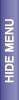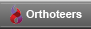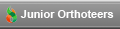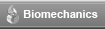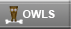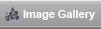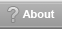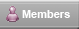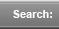# Statistics in Orthopaedics

 Data Type Description Discrete/Continuous Qualitative/Quantitative Non-Parametric/Parametric Nominal categories without order (eye colour, marital status) Discrete Qualitative N Ordinal Ordered categories (Ficat classes) Discrete Qualitative N Integer Number of counts (papers) Discrete Quantitative P/N Ratio value independent of units Continuous Quantitative P/N Interval Distances betw. units are known (hours spent studying) Continuous Quantitative P/N

Parametric Test = assume that the data were sampled from a particular form of distribution, such as a normal distribution; Non-Parametric = makes no such assumption.

Always plot data. If data is presented always ask if it has been tested for normality, skewness & kurtosis (to determine need for parametric or non-parametric analyses)

Measures of Central Tendency: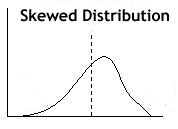• Mean - average
• Median - central value; use for ordinal data
• Mode - value with most frequency; use for nominal data

Normal (Gaussian) Distribution:

• Continuous symmetric distribution that follows the familiar bell-shaped curve
• The distribution is uniquely determined by its mean and variance
• Allows for Parametric tests (which are more powerful)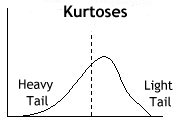• Rarely occurs in Orthopaedics
• Skewed distribution:
• Non-symmetrical, with a tail
• easily seen by plotting data
• use median or mode rather than mean
• if in doubt assume non-normality & use non-parametric tests.
• Kurtosis:
• is a measure of the heaviness of the tails in a distribution, relative to the normal distribution.
• A distribution with negative kurtosis is light-tailed relative to the normal distribution
• A distribution with positive kurtosis is heavy-tailed relative to the normal distribution

This is a preview of the site content. To view the full text for this site, you need to log in.
If you are having problems logging in, please refer to the login help page.

 © 2005-2007 Orthoteers.co.uk - last updated by Len Funk on 14 February 2005 Medical Merketing and SEO by Blue Medical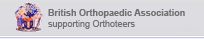Orthoteers is a non-profit educational resource. Click here for more details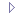Chi-Squared TestChoosing a Statistical TestCritical ReadingMeta-AnalysisStatistics examplesStatistics GlossaryStatistics in OrthopaedicsSurvivorship Analysis